March 13, 2019

## Type I and II Errors

There are two competing hypotheses: the null and the alternative. In a hypothesis test, we make a decision about which might be true, but our choice might be incorrect.

fail to reject H0 reject H0
H0 true Type I Error
HA true Type II Error

• Type I Error: Rejecting the null hypothesis when it is true.
• Type II Error: Failing to reject the null hypothesis when it is false.

## Hypothesis Test

If we again think of a hypothesis test as a criminal trial then it makes sense to frame the verdict in terms of the null and alternative hypotheses:

H0 : Defendant is innocent
HA : Defendant is guilty

Which type of error is being committed in the following circumstances?

• Declaring the defendant innocent when they are actually guilty
Type 2 error
• Declaring the defendant guilty when they are actually innocent
Type 1 error

Which error do you think is the worse error to make?

## Null Distribution

(cv <- qnorm(0.05, mean=0, sd=1, lower.tail=FALSE))
##  1.644854
PlotDist(alpha=0.05, distribution='normal', alternative='greater')
abline(v=cv, col='blue')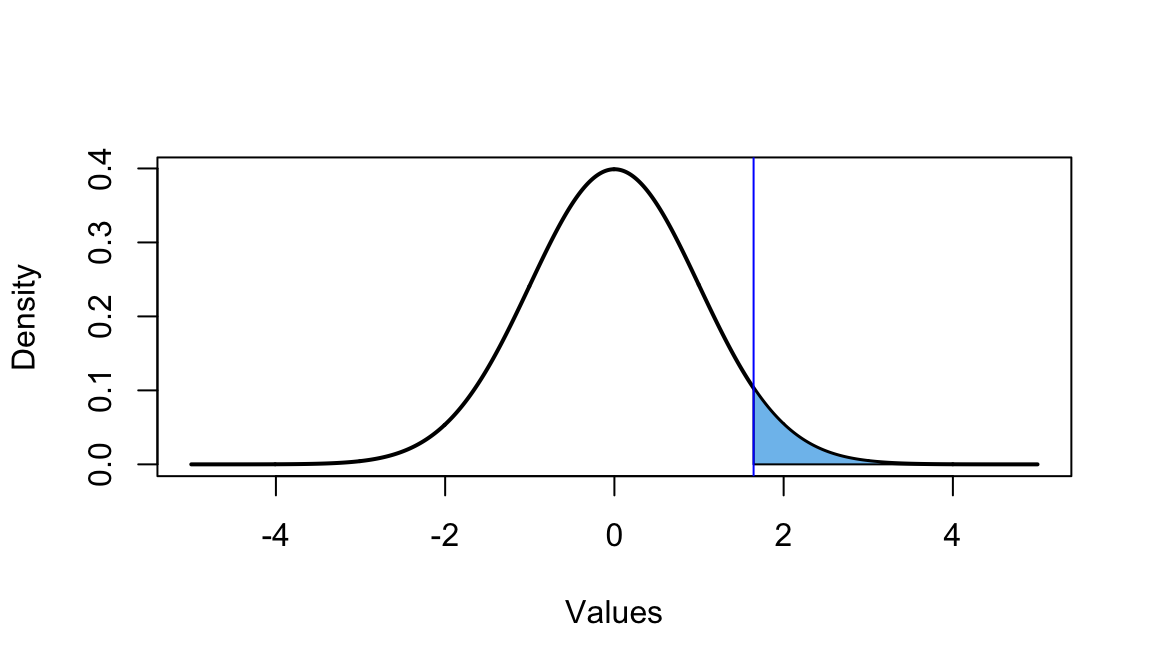## Alternative Distribution

cord.x1 <- c(-5, seq(from = -5, to = cv, length.out = 100), cv)
cord.y1 <- c(0, dnorm(mean=cv, x=seq(from=-5, to=cv, length.out = 100)), 0)
curve(dnorm(x, mean=cv), from = -5, to = 5, n = 1000, col = "black",
lty = 1, lwd = 2, ylab = "Density", xlab = "Values")
polygon(x = cord.x1, y = cord.y1, col = 'lightgreen')
abline(v=cv, col='blue')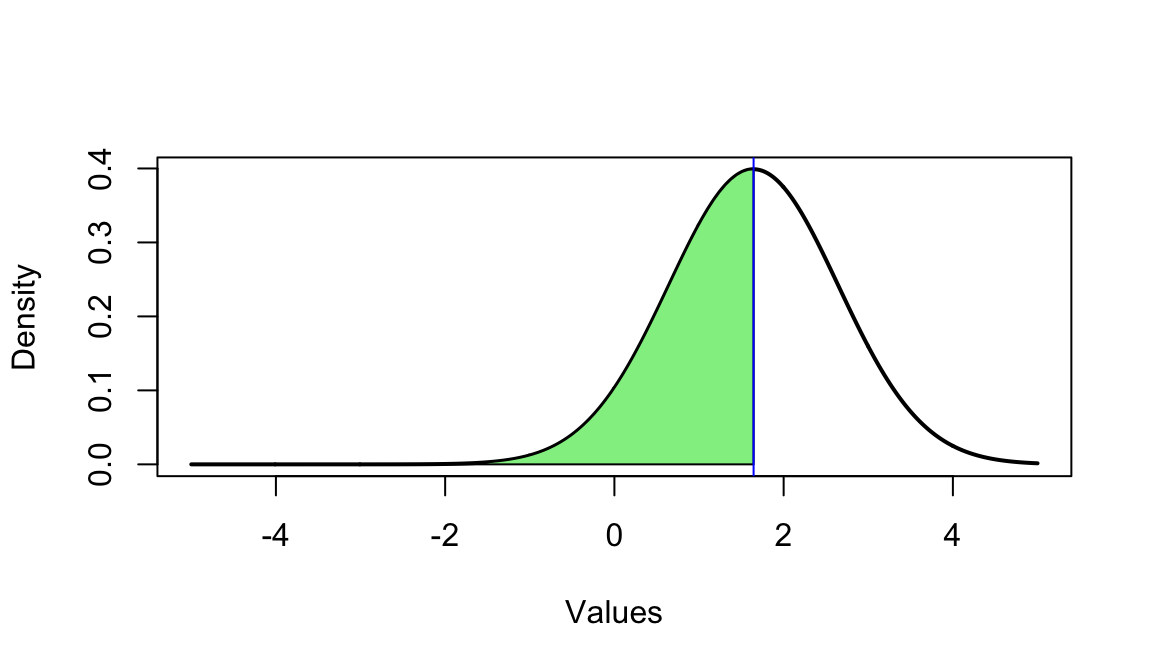pnorm(cv, mean=cv, lower.tail = FALSE)
##  0.5

## Another Example (mu = 2.5)

mu <- 2.5
(cv <- qnorm(0.05, mean=0, sd=1, lower.tail=FALSE))
##  1.644854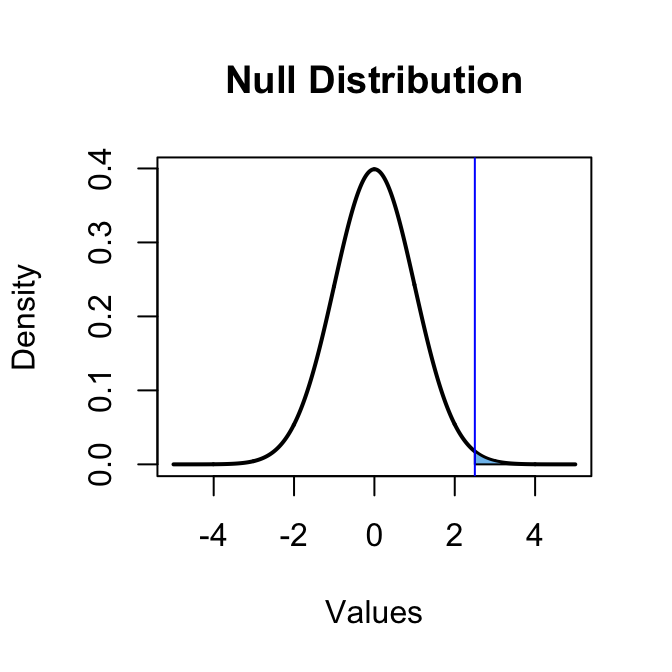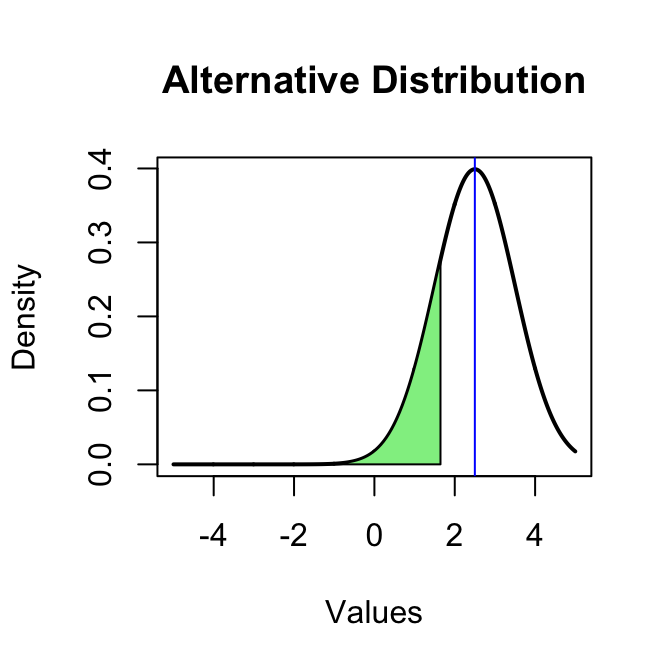## Numeric Values

Type I Error

pnorm(mu, mean=0, sd=1, lower.tail=FALSE)
##  0.006209665

Type II Error

pnorm(cv, mean=mu, lower.tail = TRUE)
##  0.1962351

## Statistical vs. Practical Significance

• Real differences between the point estimate and null value are easier to detect with larger samples.
• However, very large samples will result in statistical significance even for tiny differences between the sample mean and the null value (effect size), even when the difference is not practically significant.
• This is especially important to research: if we conduct a study, we want to focus on finding meaningful results (we want observed differences to be real, but also large enough to matter).
• The role of a statistician is not just in the analysis of data, but also in planning and design of a study.

## Bootstrapping Example (Population)

Define our population with a uniform distribution.

n <- 1e5
pop <- runif(n, 0, 1)
mean(pop)
##  0.5008915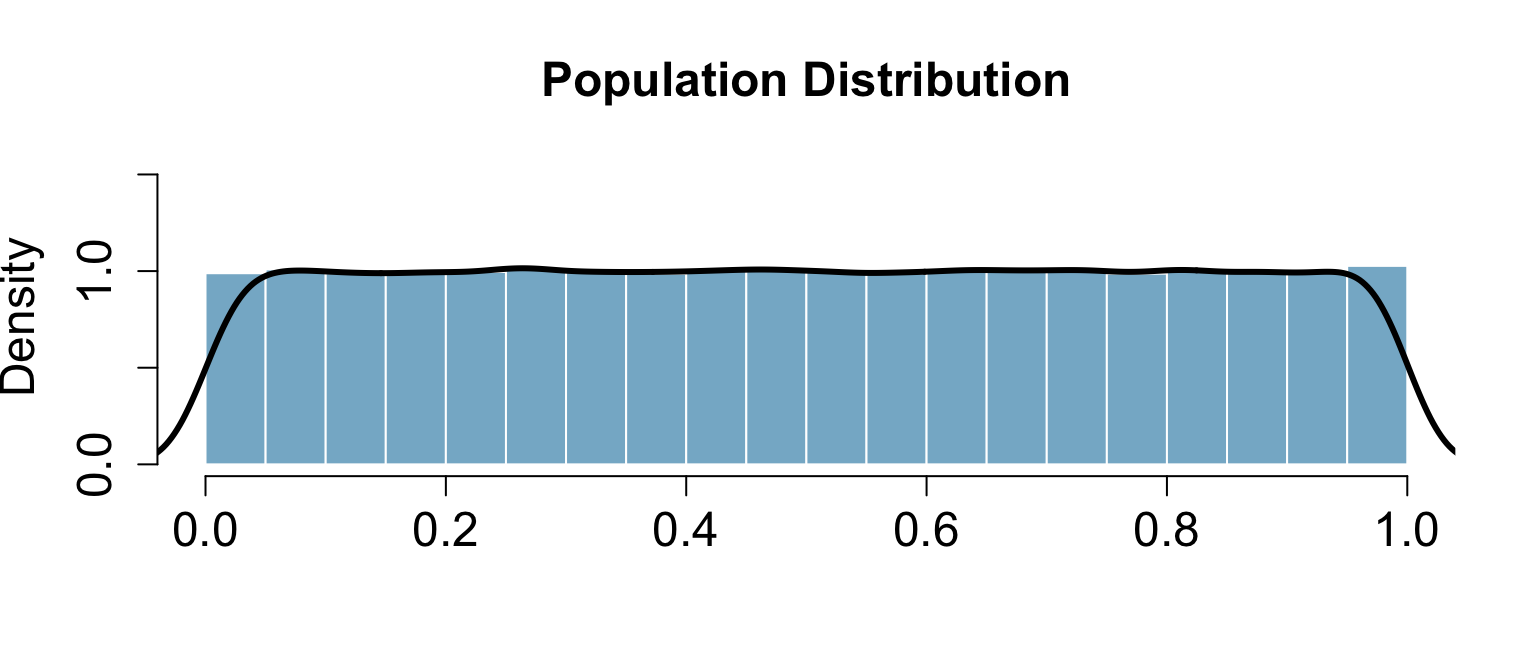## Bootstrapping Example (Sample)

We observe one random sample from the population.

samp1 <- sample(pop, size = 50)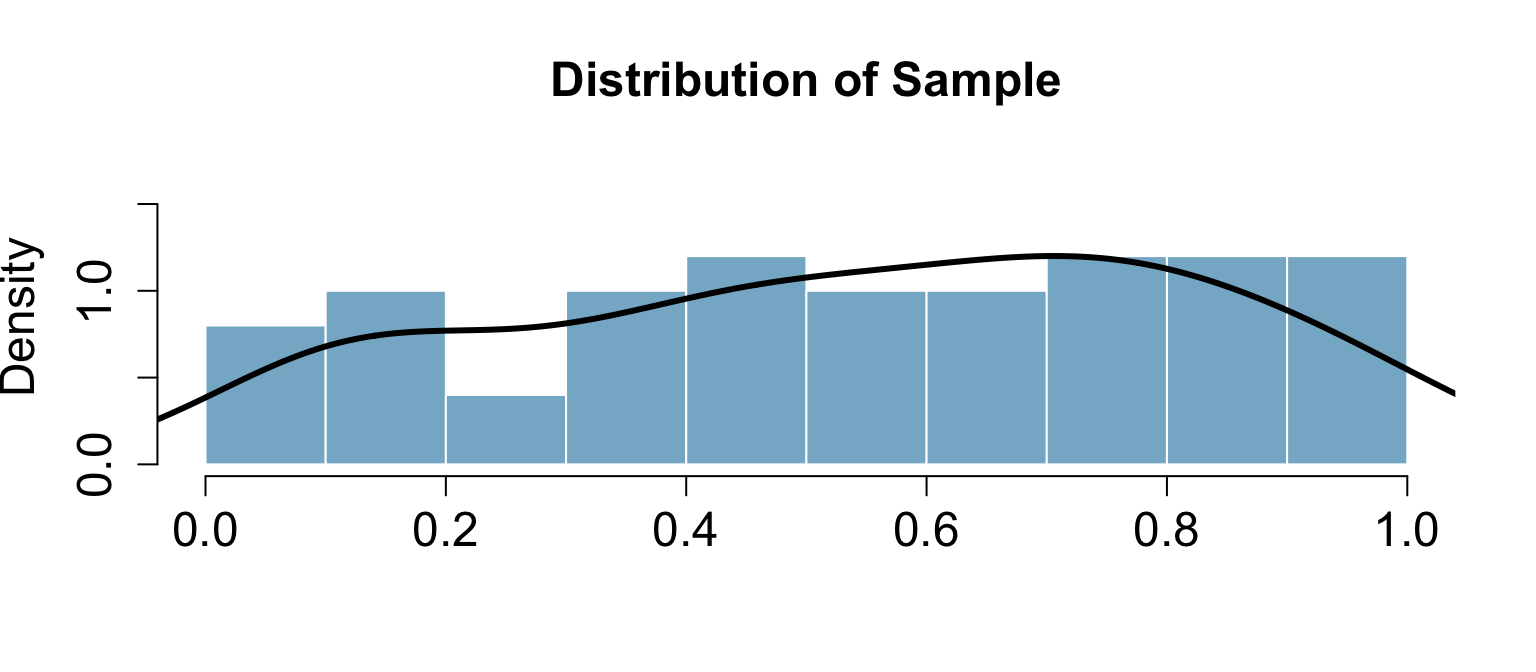## Bootsrapping Example (Estimate)

boot.samples <- numeric(1000) # 1,000 bootstrap samples
for(i in seq_along(boot.samples)) {
tmp <- sample(samp1, size = length(samp1), replace = TRUE)
boot.samples[i] <- mean(tmp)
}
head(boot.samples)
##  0.5771871 0.5807470 0.5244116 0.5417600 0.5402336 0.5336786

## Bootsrapping Example (Distribution)

d <- density(boot.samples)
h <- hist(boot.samples, plot=FALSE)
hist(boot.samples, main='Bootstrap Distribution', xlab="", freq=FALSE,
ylim=c(0, max(d$y, h$density)+.5), col=COL[1,2], border = "white",
cex.main = 1.5, cex.axis = 1.5, cex.lab = 1.5)
lines(d, lwd=3)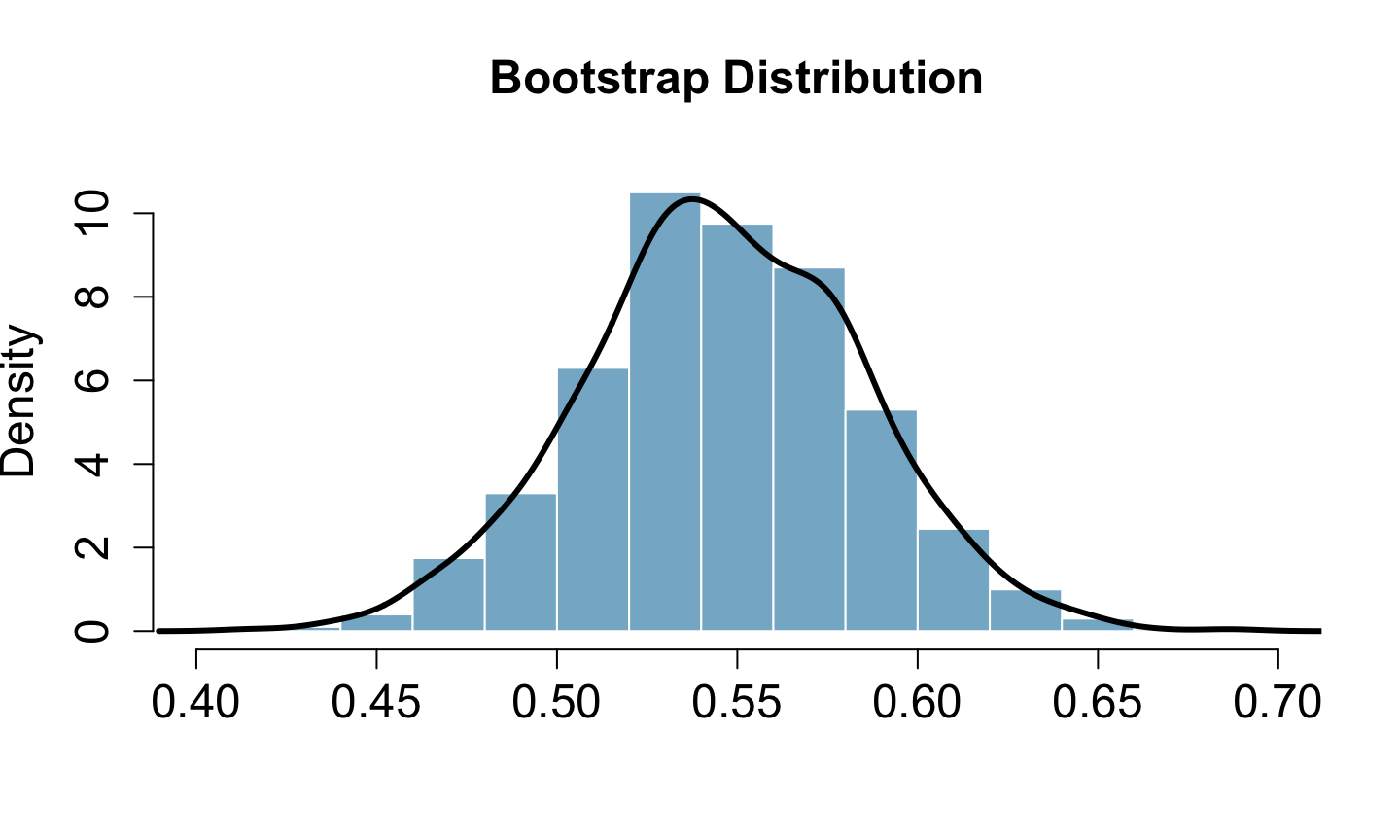## 95% confidence interval

c(mean(boot.samples) - 1.96 * sd(boot.samples),
mean(boot.samples) + 1.96 * sd(boot.samples))
##  0.4703869 0.6215272

## Bootstrapping is not just for means!

boot.samples.median <- numeric(1000) # 1,000 bootstrap samples
for(i in seq_along(boot.samples.median)) {
tmp <- sample(samp1, size = length(samp1), replace = TRUE)
boot.samples.median[i] <- median(tmp) # NOTICE WE ARE NOW USING THE median FUNCTION!
}
head(boot.samples.median)
##  0.5183295 0.5857431 0.4789167 0.6613506 0.4997448 0.5135616

95% confidence interval for the median

c(mean(boot.samples.median) - 1.96 * sd(boot.samples.median),
mean(boot.samples.median) + 1.96 * sd(boot.samples.median))
##  0.4398765 0.6971332